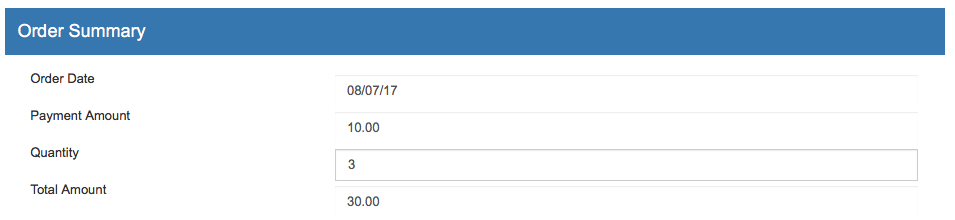Quantity

This code is for adding a quantity field to your payment form. The form takes the quantity and multiplies it by a base amount you set in the form. The below example assumes you are using the default payment form without any changes. Once the form is saved, the customer will be able to type in the quantity they are purchasing and the total will be automatically calculated. The customer will not be able to edit the amount (only the quantity). An example is shown in the image below:Javascript

Locate this section:

<script type="text/javascript">
var submitted = false;
function submitform()
{
if(submitted) {
return false;
}
submitted=true;
return true;
}
</script>

Insert a new line directly below and add the following code:

<script type="text/javascript">
{
var baseamount = document.epayform.baseamount.value ;
var quantity = document.epayform.UMquantity.value ;
var total = (baseamount*1)*quantity;
document.epayform.UMamount.value = CurrencyFormatted(total) ;
document.getElementById('totalamount').innerHTML = CurrencyFormatted(total) ;
}
</script>
<script type="text/javascript">
function CurrencyFormatted(amount)
{
var i = parseFloat(amount);
if(isNaN(i)) { i = 0.00; }
var minus = '';
if(i < 0) { minus = '-'; }
i = Math.abs(i);
i = parseInt((i + .005) * 100);
i = i / 100;
s = new String(i);
if(s.indexOf('.') < 0) { s += '.00'; }
if(s.indexOf('.') == (s.length - 2)) { s += '0'; }
s = minus + s;
return s;
}
</script>

HTML

Find and delete the following code in your form:

<input type="hidden" name="UMamount" value="[UMamount]">

Find the following code in your form:

<div class="form-group">
<span class="col-form-label col-sm-4 col-xs-12 ">Order Amount</span>
<div class="col-md-8 col-xs-12 ">
<input id="" name="UMamount" class="form-control" type="text" placeholder="Order Amount" value="[UMamount]">
</div>
</div>

Replace it with the following. For this example, the UMamout for the product is 10.00 (or \$10). Remember to replace 10.00 with the correct amount for your needs before pasting into your form:

<div class="form-group">
<span class="col-form-label col-sm-4 col-xs-12 ">Payment Amount</span>
<div class="col-md-8 col-xs-12 ">
</div>
</div>
<div class="form-group">
<span class="col-form-label col-sm-4 col-xs-12 ">Quantity</span>
<div class="col-md-8 col-xs-12 ">
<input id="" name="UMquantity" class="form-control" type="text" placeholder="Quantity" onChange="addCharge()">
</input>
</div>
</div>
<div class="form-group">
<span class="col-form-label col-sm-4 col-xs-12 ">Total Amount</span>
<div class="col-md-8 col-xs-12 ">